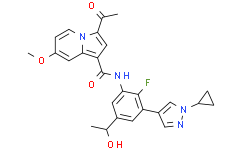# Y-08284

Cat No.:V50812 Purity: ≥98%
Y-08284, CBP bromodomain inhibitorY-08284 Chemical Structure CAS No.: 2688745-47-1
Product category: New3
This product is for research use only, not for human use. We do not sell to patients.
Size Price
100mg
250mg
500mgTop Publications Citing lnvivochem Products
Product Description
Y-08284, CBP bromodomain inhibitor
Physicochemical Properties
Molecular Formula C26H25FN4O4 476.499509572983 2688745-47-1 C1(C(NC2=CC(C(O)C)=CC(C3=CN(C4CC4)N=C3)=C2F)=O)=C2N(C=CC(OC)=C2)C(C(C)=O)=C1 Powder      -20°C    3 years                      4°C     2 years In solvent   -80°C    6 months                   -20°C    1 month Room temperature (This product is stable at ambient temperature for a few days during ordinary shipping and time spent in Customs)
Solubility Data
 Preparing Stock Solutions 1 mg 5 mg 10 mg 1 mM 2.0986 mL 10.4932 mL 20.9864 mL 5 mM 0.4197 mL 2.0986 mL 4.1973 mL 10 mM 0.2099 mL 1.0493 mL 2.0986 mL

*Note: Please select an appropriate solvent for the preparation of stock solution based on your experiment needs. For most products, DMSO can be used for preparing stock solutions (e.g. 5 mM, 10 mM, or 20 mM concentration); some products with high aqueous solubility may be dissolved in water directly. Solubility information is available at the above Solubility Data section. Once the stock solution is prepared, aliquot it to routine usage volumes and store at -20°C or -80°C. Avoid repeated freeze and thaw cycles.

Calculator

### Molarity Calculator allows you to calculate the mass, volume, and/or concentration required for a solution, as detailed below:

• Calculate the Mass of a compound required to prepare a solution of known volume and concentration
• Calculate the Volume of solution required to dissolve a compound of known mass to a desired concentration
• Calculate the Concentration of a solution resulting from a known mass of compound in a specific volume
An example of molarity calculation using the molarity calculator is shown below:
What is the mass of compound required to make a 10 mM stock solution in 5 ml of DMSO given that the molecular weight of the compound is 350.26 g/mol?
• Enter 350.26 in the Molecular Weight (MW) box
• Enter 10 in the Concentration box and choose the correct unit (mM)
• Enter 5 in the Volume box and choose the correct unit (mL)
• Click the “Calculate” button
• The answer of 17.513 mg appears in the Mass box. In a similar way, you may calculate the volume and concentration.

### Dilution Calculator allows you to calculate how to dilute a stock solution of known concentrations. For example, you may Enter C1, C2 & V2 to calculate V1, as detailed below:

What volume of a given 10 mM stock solution is required to make 25 ml of a 25 μM solution?
Using the equation C1V1 = C2V2, where C1=10 mM, C2=25 μM, V2=25 ml and V1 is the unknown:
• Enter 10 into the Concentration (Start) box and choose the correct unit (mM)
• Enter 25 into the Concentration (End) box and select the correct unit (mM)
• Enter 25 into the Volume (End) box and choose the correct unit (mL)
• Click the “Calculate” button
• The answer of 62.5 μL (0.1 ml) appears in the Volume (Start) box
g/mol

### Molecular Weight Calculator allows you to calculate the molar mass and elemental composition of a compound, as detailed below:

Note: Chemical formula is case sensitive: C12H18N3O4  c12h18n3o4
Instructions to calculate molar mass (molecular weight) of a chemical compound:
• To calculate molar mass of a chemical compound, please enter the chemical/molecular formula and click the “Calculate’ button.
Definitions of molecular mass, molecular weight, molar mass and molar weight:
• Molecular mass (or molecular weight) is the mass of one molecule of a substance and is expressed in the unified atomic mass units (u). (1 u is equal to 1/12 the mass of one atom of carbon-12)
• Molar mass (molar weight) is the mass of one mole of a substance and is expressed in g/mol.
/

### Reconstitution Calculator allows you to calculate the volume of solvent required to reconstitute your vial.

• Enter the mass of the reagent and the desired reconstitution concentration as well as the correct units
• Click the “Calculate” button
• The answer appears in the Volume (to add to vial) box
In vivo Formulation Calculator (Clear solution)
Step 1: Enter information below (Recommended: An additional animal to make allowance for loss during the experiment)
Step 2: Enter in vivo formulation (This is only a calculator, not the exact formulation for a specific product. Please contact us first if there is no in vivo formulation in the solubility section.)
+
+
+

Calculation results

Working concentration mg/mL；

Method for preparing DMSO stock solution mg drug pre-dissolved in μL DMSO (stock solution concentration mg/mL). Please contact us first if the concentration exceeds the DMSO solubility of the batch of drug.

Method for preparing in vivo formulation:Take μL DMSO stock solution, next add μL PEG300, mix and clarify, next addμL Tween 80, mix and clarify, next add μL ddH2O，mix and clarify.

(1) Please be sure that the solution is clear before the addition of next solvent. Dissolution methods like vortex, ultrasound or warming and heat may be used to aid dissolving.
(2) Be sure to add the solvent(s) in order.

Related Products